# Smooth Binary Image Before Surface Extraction#

## Synopsis#

This example introduces the use of the AntiAliasBinaryImageFilter. This filter expects a binary mask as input. With level sets, it smooths the image by keeping the edge of the structure within a one pixel distance from the original location. It is usually desirable to run this filter before extracting an isocontour with surface extraction methods.

## Code#

### Python#

#!/usr/bin/env python

import itk
import argparse

parser = argparse.ArgumentParser(
description="Smooth Binary Image Before Surface Extraction."
)
args = parser.parse_args()

PixelType = itk.F
Dimension = 2
ImageType = itk.Image[PixelType, Dimension]

AntiAliasFilterType = itk.AntiAliasBinaryImageFilter[ImageType, ImageType]
antialiasfilter = AntiAliasFilterType.New()
antialiasfilter.SetMaximumRMSError(args.maximum_RMS_error)
antialiasfilter.SetNumberOfIterations(args.number_of_iterations)
antialiasfilter.SetNumberOfLayers(args.number_of_layers)

WriterType = itk.ImageFileWriter[ImageType]
writer = WriterType.New()
writer.SetFileName(args.output_image)
writer.SetInput(antialiasfilter.GetOutput())

writer.Update()


### C++#

#include "itkImageFileReader.h"
#include "itkImageFileWriter.h"
#include "itkAntiAliasBinaryImageFilter.h"

int
main(int argc, char * argv[])
{
if (argc < 3)
{
std::cerr << "Usage: " << std::endl;
std::cerr << argv << " <inputImage> <outputImage>";
std::cerr << " [maximumRMSError]";
std::cerr << " [numberOfIterations]";
std::cerr << " [numberOfLayers]" << std::endl;
return EXIT_FAILURE;
}

const char * inputFileName = argv;
const char * outputFileName = argv;
double       maximumRMSError = 0.001;
if (argc > 3)
{
maximumRMSError = std::stod(argv);
}
int numberOfIterations = 50;
if (argc > 4)
{
numberOfIterations = std::stoi(argv);
}
int numberOfLayers = 2;
if (argc > 5)
{
numberOfLayers = std::stoi(argv);
}

constexpr unsigned int Dimension = 2;
using PixelType = float;
using ImageType = itk::Image<PixelType, Dimension>;

using FilterType = itk::AntiAliasBinaryImageFilter<ImageType, ImageType>;
auto filter = FilterType::New();
filter->SetInput(input);
filter->SetMaximumRMSError(maximumRMSError);
filter->SetNumberOfIterations(numberOfIterations);
filter->SetNumberOfLayers(numberOfLayers);

try
{
itk::WriteImage(filter->GetOutput(), outputFileName);
}
catch (const itk::ExceptionObject & error)
{
std::cerr << "Error: " << error << std::endl;
return EXIT_FAILURE;
}

return EXIT_SUCCESS;
}


## Classes demonstrated#

template<typename TInputImage, typename TOutputImage>
class AntiAliasBinaryImageFilter : public itk::SparseFieldLevelSetImageFilter<TInputImage, TOutputImage>

A method for estimation of a surface from a binary volume.

This filter implements a surface-fitting method for estimation of a surface from a binary volume. This process can be used to reduce aliasing artifacts which result in visualization of binary partitioned surfaces.

The binary volume (filter input) is used as a set of constraints in an iterative relaxation process of an estimated ND surface. The surface is described implicitly as the zero level set of a volume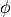and allowed to deform under curvature flow. A set of constraints is imposed on this movement as follows: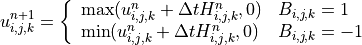where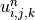is the value ofat discrete index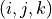and iteration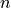,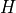is the gradient magnitude times mean curvature of, and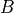is the binary input volume, with 1 denoting an inside pixel and -1 denoting an outside pixel.

NOTES

This implementation uses a sparse field level set solver instead of the narrow band implementation described in the reference below, which may introduce some differences in how fast and how accurately (in terms of RMS error) the solution converges.

REFERENCES

Whitaker, Ross. “Reducing Aliasing Artifacts In Iso-Surfaces of Binary

Volumes” IEEE Volume Visualization and Graphics Symposium, October 2000, pp.23-32.

PARAMETERS

The MaximumRMSChange parameter is used to determine when the solution has converged. A lower value will result in a tighter-fitting solution, but will require more computations. Too low a value could put the solver into an infinite loop. Values should always be less than 1.0. A value of 0.07 is a good starting estimate.

The MaximumIterations parameter can be used to halt the solution after a specified number of iterations.

INPUT

The input is an N-dimensional image of any type. It is assumed to be a binary image. The filter will use an isosurface value that is halfway between the min and max values in the image. A signed data type is not necessary for the input.

OUTPUT

The filter will output a level set image of real, signed values. The zero crossings of this (N-dimensional) image represent the position of the isosurface value of interest. Values outside the zero level set are negative and values inside the zero level set are positive values.

IMPORTANT!

The output image type you use to instantiate this filter should be a real valued scalar type. In other words: doubles or floats.

USING THIS FILTER

The filter is relatively straightforward to use. Tests and examples exist to illustrate. The important thing is to understand the input and output types so you can properly interpret your results.

In the common case, the only parameter that will need to be set is the MaximumRMSChange parameter, which determines when the solver halts.

ITK Sphinx Examples: